Case with two camerasNext: Mosaics Up: Mosaic Tutorial Previous: Projective formalism

# Perspective projection into two views

Let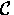,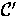, be two cameras, with respective optical centers C,C', we associate the frameof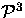to the cameraand the frame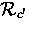ofto the camera.

Let M be a point of the projective space, represented inby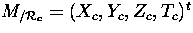and inby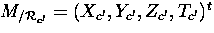, we call m and m' their two respective projections in the frame induced byin the focal plane of the camera, and byin the focal plane of the camera.

the relation (1) can be written for the point m':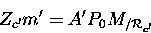(6)

we have also: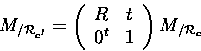(7)

combining (6) and (7) and doing some calculus we obtain the relation 8: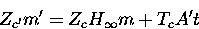(8)

where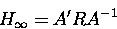(9)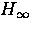is the homography of the infinite plane. Note that if t=0 (i.e. we have no translation between the two views, but only rotation), the relation 9 will become a homographic relation (i.e. relation in the projective plane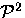).

Obviously, if the points are coplanar we have also a homographic relation between the points. In formula 10 we present the relation in case of co-planarity (we obtain this relation starting from formula 8 and doing some simplification using the co-planarity of the points).

 Zc'm' = Zc H m (10)

where: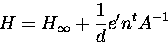(11)Next: Mosaics Up: Mosaic Tutorial Previous: Projective formalism